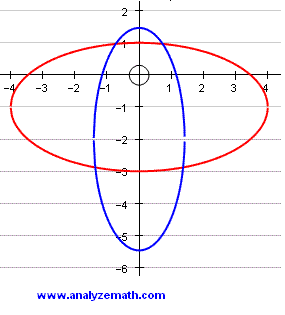# Find the Points of Intersection of Two Ellipses

This is tutorial on finding the points of intersection of two ellipses given by their equations.

### Example 1

Find the points of intersection of the two ellipses given by their equations as follows:
x2 / 16 + (y + 1)2 / 4 = 1
x2 / 2 + (y + 2)2 / 12 = 1
Solution to Example 1:

We first multiply all terms of the first equation by 16 and all the terms of the second equation by - 2 to obtain equivalent equations:
x2 + 4 (y + 1)2 = 16
- x2 - (1/6) (y + 2)2 = - 2
We now add the same sides of the two equations to obtain a quadratic equation
4 (y + 1)2 - (1 / 6) (y + 2)2 = 14
Multiply all terms by 6, group like terms and rewrite the equation as
23 y2 + 44y - 64 = 0
Solve the quadratic equation for y to obtain two solutions
y ≈ 0.97 and y ≈ -2.88
We now substitute the values of y already obtained into the equation x2 + 4 (y + 1)2 = 16 and solve it for x to obtain the x values
for y ≈ 0.97 ; x values are given by: x ≈ 0.730365 and x ≈ -0.730365
for y ≈ -2.88 ; x values are given by: x ≈ 1.36788 and x ≈ -1.36788
The 4 points of intersection of the two ellipses are
( 0.730365 , 0.97) ; ( -0.73 , 0.97) ; (1.37 , -2.88) ; (- 1.36788 , -2.88)
The graph of the two ellipses given above by their equations are shown below with their points of intersection.### More Links and References on Ellipses

Points of Intersection of an Ellipse and a line
Find the Points of Intersection of a Circle and an Ellipse
Equation of Ellipse, Problems.
College Algebra Problems With Answers - sample 8: Equation of Ellipse
HTML5 Applet to Explore Equations of Ellipses
Ellipse Area and Perimeter Calculator